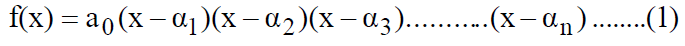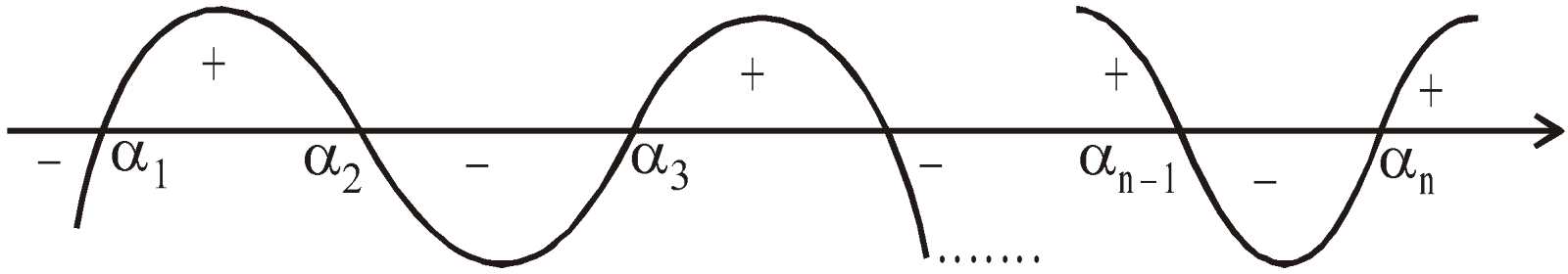# LINEAR INEQUALITIES

### INTERVAL NOTATION IN REAL NUMBERS

Let a and b be two given numbers such that a < b. The set of numbers x, lying between these numbers a and b may be represented in a number of ways as following :

#### CLOSED INTERVAL

The set of numbers x such that a ≤ x ≤ b is a closed Interval, denoted by [a, b], henceThus [a, b] contains all real numbers between the numbers a and b including the numbers a and b too.

#### OPEN INTERVAL

The set of numbers x, such that a < x < b   is called an open Interval, denoted by (a, b), henceThus (a, b) contains all numbers between the numbers a and b however excluding a and b.

#### SEMI-OPEN OR SEMI-CLOSED INTERVAL

Semi intervals may be defined as follows:, includes b but excludes a, excludes b but includes a

REMARKS
• The number 'b – a' is referred to as the length of any of the above intervals.
• Let a be a real number then the set of all the real numbers x > a are denoted byandis denoted by• Similarly if b is a real number, then x < b is equivalent toandis equivalent to### MODULUS OF A REAL NUMBERS

Let x be a real number then it may be a positive number, a negative number or zero. By modulus of x (denoted by, read as "mod x") we mean the absolute value of the number x, i.e., its magnitude only without any consideration to sign. We defineExample :
| 3 |    = 3, as 3 > 0,
| 0 |    = 0
| -2 | =  –(–2) = 2, as –2 < 0
Hence the above definition makes always nonnegative, whether x is positive or negative. We may give following alternate representations
| x |  = max {x, -x}
| x |#### PROPERTIES OF MODULUS

Let x and y be real numbers then
1. | x |, i.e., modulus of a real number is always non-negative
2. | x | > x,3.4.In inequalities 3 and 4 equality holds if and only if
x.y > 0.
1.2.3. Ifthenwhere 'a' is a nonnegative real number
4. If | x | < a, then – a < x < a, where a is a  positive real number.
5. If | x | > a, then x < –a or x > a, where a is a non-negative real number.

### MODULUS OF A FUNCTION

Let f(x) is a function of real number x, thenExample :
1.1.1.Students have a common tendency of applying the conditionsandon every situation. Note that these conditions were applied basically to define absolute value of the number x. Hence in a problem of the type containing, one must apply conditionsand(see above examples). See the illustrative examples for better understanding.

### GENERAL THEORY OF POLYNOMIAL EQUATIONS

Consider the equation.
The above equation (1) is a polynomial equation of nth degree. It has exactly n roots. Let the roots of equation (1) bethenandFor example :
If α, β, γ be the roots of cubic equation  ax3 + bx2 + cx + d = 0.
Then;;Ifbe the roots of bi-quadratic equation
ax4 + bx3 + cx2 + dx + e = 0
Then;;Note : The general quadratic expression ax2 + 2hxy + by2 + 2gx + 2fy + c in x and y may be resolved into two linear rational factors if abc + 2fgh – af2 – bg2 –ch2 = 0
or### SOLVING INEQUALITIES USING METHOD OF INTERVALS (WAVY CURVE METHOD)

Consider a polynomial expression f(x) of n degree, expressed in terms of linear factors :LetTo determine the sign of f(x), we may draw the following curve+ and – signs represent the sign scheme for f(x) in various intervals. We draw the above curve from above the real line right to left alternatively changing the curve at points### RATIONAL INEQUALITIES

Consider the inequalityThe above inequality is solved by multiplying numerator and denominator by g(x),
We getandThe above inequality can now be solved by using method of intervals.

### LOGARITHMIC EQUATIONS AND INEQUALITIES

If ab = c, then b is said to be the logarithm of c to the base a and we write. Where,#### LAWS OF LOGARITHMS

1.2.3.4.5.6.7.8.9.#### SOLVING LOGARITHMIC EQUATION

1. Ifwhere2. If3. If#### SOLVING LOGARITHMIC INEQUALITIES

1. Ifif 0 < a < 1
2. Ifif a > 1 and x > y if 0 < a < 1

### EQUATION AND INEQUALITIES CONTAINING GREATEST INTEGER FUNCTION [X]

Let. x can always be expressed as a sum of an integer and a pure fraction. Thus,
x = I + f, where I is an integer andI is called integral part of x and f is fractional part of x.
We often denote I by [x] and f by {x}
∴     [x] = the integral part of x
= the greatest integer less than or equal to x
and {x} = the fractional part of x

#### PROPERTIES OF [X]

1. x = [x] + {x}
2.3.4.5.6.7.8. For any x  ∈ R and n ∈ NExample:## Want to know more

Please fill in the details below:

## Latest NEET Articles\$type=three\$c=3\$author=hide\$comment=hide\$rm=hide\$date=hide\$snippet=hide

Name

ltr
item
BEST NEET COACHING CENTER | BEST IIT JEE COACHING INSTITUTE | BEST NEET, IIT JEE COACHING INSTITUTE: Linear Inequalities | Mathematics Notes for IITJEE Main
Linear Inequalities | Mathematics Notes for IITJEE Main